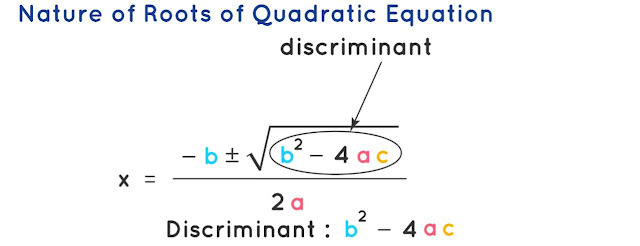# Quadratic Equation Class 11 Mathematics Solutions | Exercise - 6.2## Chapter - 6  Quadratic Equation

An Equation of second degree is known as Quadratic Equation. It means the highest exponent of this function is 2. The standard form of a quadratic equation is y = ax²+ bx + c, where a, b, and c are numbers and a cannot be 0. Examples of quadratic equations include all of these: y = x²+ 4x + 2, y = 2x² + 4x - 9, y= x² etc.

In this chapter, we will learn different theorems which will provide us clear understanding about the terms related to quadratic equation. It is better to learn some formulas and important topics related to quadratic equation before moving towards the solution section. So let's have a brief introduction of the quadratic equation chapter.

### Introduction

In algebra, a quadratic equation is any equation that can be rearranged in standard form y = ax²+ bx + c, where x represents an unknown value, and a, b, and c represent known numbers, where a ≠ 0. If b = 0, the equation is known as the pure quadratic equation and if b ≠ 0, then the equation is known as the adfected quadratic

### Symmetric Function of Roots

Symmetric functions of the roots of a quadratic equation are those functions in which the two roots are so involved that the function is unaltered when the two roots are interchanged. For instance, the sum α+β and the product αβ of the roots, we have considered in the last two sections, are symmetric functions of α and β.

### Sum and Product of Roots of Quadratic Equation

The coefficient of x², x term, and the constant term of the quadratic equation ax²+ bx + c = 0 are very useful in determining the sum and product of the roots of the quadratic equation. It can be directly calculated from the equation, without actually finding the roots of the quadratic equation. For a quadratic equation ax² + bx + c = 0, the sum and product of the roots are as follows.

• Sum of the Roots: α + β = -b/a = - Coefficient of x/ Coefficient of x²
• Product of the Roots: αβ = c/a = Constant term/ Coefficient of x²### Exercise - 6.2

In this PDF, you'll only find the solution of class 11 quadratic equation chapter. It contains all the solutions of exercise- 5.2. If you want the solutions of other exercises then you'll find them above this PDF. Just click on the button and you'll reach your destination.

NoteScroll the PDF to view all Solution

You are not allowed to post this PDF in any website or social platform without permission.

## Is Class 11 Mathematics Guide Helpful For Student ?

I have published this Notes for helping students who can't solve difficult maths problems. Student should not fully depend on this note for completing all the exercises. If you totally depend on this note and simply copy as it is then it may affect your study.

Student should also use their own will power and try to solve problems themselves. You can use this mathematics guide PDF as a reference. You should check all the answers before copying because all the answers may not be correct. There may be some minor mistakes in the note, please consider those mistakes.

## How to secure good marks in Mathematics ?

As, you may know I'm also a student. Being a student is not so easy. You have to study different subjects simultaneously. From my point of view most of the student are weak in mathematics. You can take me as an example, I am also weak in mathematics. I also face problems while solving mathematics questions.

If you want to secure good marks in mathematics then you should practise them everyday. You should once revise all the exercise which are already taught in class. When you are solving maths problems, start from easy questions that you know already. If you do so then you won't get bored.

Maths is not only about practising, especially in grade 11 you to have the basic concept of the problem. When you get the main concept of the problem then you can easily any problems in which similar concept are applied.

When your teacher tries to make the concept clear by giving examples then all students tries to remember the same example but you should never do that. You can create your own formula which you won't forget later.

If you give proper time for your practise with proper technique then you can definitely score a good marks in your examination.

Disclaimer: This website is made for educational purposes. If you find any content that belongs to you then contact us through the contact form. We will remove that content from our as soon as possible.

If you have any queries then feel free to comment down.
No Comment